# Resolution of the identity

(diff) ← Older revision | Latest revision (diff) | Newer revision → (diff)

A one-parameter family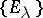,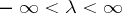, of orthogonal projection operators acting on a Hilbert space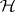, such that

1)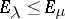if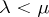;

2)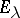is strongly left continuous, i.e.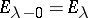for every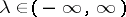;

3)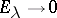as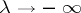and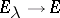as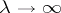; here 0 andare the zero and the identity operator on the space.

Condition 2) can be replaced by the condition of strong right continuity at every point.

Every self-adjoint operatoracting ongenerates in a unique way a resolution of the identity. Here, in addition to 1)–3), the following conditions also hold:

4) ifis a bounded operator such that, thenfor any;

5) ifis a bounded operator and,are its greatest lower and least upper bounds, respectively, then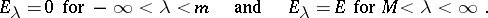The resolution of the identity given by the operatorcompletely determines the spectral properties of that operator, namely:

a) a pointis a regular point ofif and only if it is a point of constancy, that is, if there is asuch that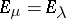for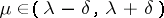;

b) a pointis an eigenvalue ofif and only if at this pointhas a jump, that is,;

g) if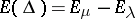, then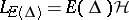is an invariant subspace of.

Hence the resolution of the identity determined by the operatoris also called the spectral function of this operator (cf. Spectral resolution).

Conversely, every resolution of the identityuniquely determines a self-adjoint operatorfor which this resolution is the spectral function. The domain of definitionofconsists exactly of thosefor which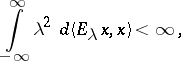and there is a representation ofas an operator Stieltjes integral:How to Cite This Entry:
Resolution of the identity. Encyclopedia of Mathematics. URL: http://encyclopediaofmath.org/index.php?title=Resolution_of_the_identity&oldid=13743
This article was adapted from an original article by V.I. Sobolev (originator), which appeared in Encyclopedia of Mathematics - ISBN 1402006098. See original article